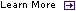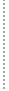Construction Audits Let our Experts find overcharges and reduce your risks.Lease Audits We will ensure you are not overpaying your Landlord.Cost Segregation Study Our Engineers will maximize your tax benefits.Construction Consulting Effective Cost Control and Process Improvement strategies.Statistical Sampling Statistical Sampling   /  irs audit techniques guide  /  cost segregation study   /  www.commercialcostcontrol.com

 Related Content## Cost Segregation ATG - Chapter 6.5 Statistical Sampling

 Note: Each chapter of this Audit Techniques Guide (ATG) can be printed individually. Please follow the links at the beginning or end of this chapter to return to either the previous chapter or the Table of Contents or to proceed to the next chapter.APPENDIX - CHAPTER 6.5 - STATISTICAL SAMPLINGA memorandum on March 14, 2002 (which follows) was issued to provide field guidance on statistical sampling. Examiners can also contact their local Computer Audit Specialist (CAS) for assistance. Note that Attachment A contains complex formulas and can be viewed at the following link: http://www.irs.gov/pub/irs-utl/dir_use_prob_sampling.pdfMarch 14, 2002MEMORANDUM FOR INDUSTRY DIRECTORS, LMSBDIRECTOR, PRE-FILING & TECHNICAL GUIDANCE, LMSBFROM:          Keith M. JonesDirector, Field SpecialistsSUBJECT:     Field Directive on the Use of Estimates from Probability SamplesThe purpose of this memorandum is to establish guidelines for the Internal Revenue Service in evaluating samples and sampling estimates by taxpayers. These guidelines are intended to promote efficiency and consistency of the probability samples performed and examined by the IRS. They are not intended to be a technical position but to provide audit issue direction to effectively utilize our resources. Further, as more fully described below, they are not intended to replace or supersede specific statutory or regulatory requirements for substantiation or record keeping.Examiners should perform a two-step inquiry in evaluating a taxpayers probability sample. First, they should determine whether the taxpayer has appropriately used a probability sample to support or be the primary evidence of tax amounts. Second, they should determine whether the final answer represents a valid estimate.The appropriateness of using a probability sample is a facts and circumstances determination. Some of the factors to be used in determining whether a probability sample is appropriate include the time required to analyze large volumes of data, the cost of analyzing data, and other books and records that may independently exist or have greater probative value.back to the top Probability samples generally should be considered appropriate if there is a compelling reason for their use and taxpayers cannot reasonably obtain more accurate information. However, probability samples generally should not be considered appropriate if evidence is readily available from another source that can be demonstrated to be a more accurate answer, or if the use of sampling does not conform to Generally Accepted Accounting Principles (GAAP).Once examiners determine that the use of a probability sample is appropriate, they should determine the validity of the final estimate. In general, an estimate from a taxpayers sample should be considered valid (without regard to adjustment(s) based on audit issues) if all of the following conditions are met.The taxpayer has maintained all of the proper documentation to support the statistical application, sample unit findings and all aspects of the sample plan. This will generally include all of the information contained in Attachment A to these guidelines. The documentation requirement helps insure that the sample was conducted in a manner to support all the necessary elements of a probability sample.The estimate is based on a probability (i.e., statistical) sample, where each sampling unit has a known (non-zero) chance of selection, using either a simple random sampling method or stratified random sampling method.The estimate is computed at the least advantageous 95% one-sided confidence limit. The "least advantageous" confidence limit is either the upper or lower limit that results in the least benefit to the taxpayer. Recognizing that many methods exist to estimate population values from the sample data, only the following estimators will be considered for acceptance. Variable estimators permitted include the Mean (also known as the direct projection method), Difference (using "paired variables"), (combined) Ratio (using a variable of interest and a "correlated" variable), and (combined) Regression (using a variable of interest and a "correlated" variable).1 Since the latter two variable methods are statistically biased, it must be demonstrated that such bias is negligible before they will be considered acceptable. The formulas for these estimators are in the Technical Appendix to these guidelines and assume sampling without replacement. Attribute estimators permitted include (combined) proportion or total count.Variable Sampling Plans.Of all the final estimates determined as qualifying, the estimate with the smallest overall standard error, as an absolute value, must be used (i.e., the size of the estimate is irrelevant in the determination of the value to be reported).Confidence limits are calculated by adding and subtracting the precision of the estimate from the point estimate where precision is determined by multiplying the standard error by (i) the 95% one-sided confidence coefficient based on the Students t-distribution with the appropriate degrees of freedom, or (ii) 1.645 (i.e., the normal distribution), assuming the sample size is at least 100 in each non-100% stratum.For either the (combined) Ratio or Regression methods, to demonstrate little statistical bias exists, the following applies after excluding all strata tested on a 100% basis (i.e., the entire population of a stratum is selected for evaluation).The total sample size of all strata must be at least 100 units.Each stratum for which a population estimate is made should contain at least 30 sample units.The coefficient of variation of the paired variable2 must be 15% or less.The coefficient of variation of the primary variable of interest, represented by either the corrected value3 or the difference between the reported and corrected values4 in common accounting situations, must be 15% or less.For only the (combined) Ratio method the reported values of the units must be of the same sign.back to the topAttribute Sampling Plans:When using simple random samples, the confidence limits will be determined using the Hypergeometric, Poisson, or Binomial distribution. If the proportion being estimated is between 30% and 70%, then the normal distribution approximation may be used in lieu of one of the above distributions.For stratified random samples, when at least two strata are sampled (i.e., not 100% samples), the confidence limits must be determined using the normal distribution approximation. Otherwise, item one above applies.For the normal distribution approximation, the precision is calculated by multiplying the standard error by (i) the 95% one-sided confidence coefficient based on the Students t-distribution with the appropriate degrees of freedom, or (ii) 1.645 (i.e., the normal distribution), assuming the sample size is at least 100 in each non-100% stratum.The allowance of a taxpayers estimate does not correspondingly require acceptance of the taxpayers use of such estimate for the determination of associated adjustments, allocation, or subdivision of the findings for other purposes unless statistically determined according to these guidelines and applied on a basis appropriate for the circumstances. These guidelines address only the statistical requirements that must be met for a probability sample to meet preliminary acceptance and are not intended to further require acceptance of individual sample unit determinations. Valuation or attribute determinations remain subject to independent verification along with other non-statistical issues such as missing sampling items. Likewise, the statistical procedures followed may be examined and adjusted when discovered in error. Corrections to statistical methodology are permitted where possible to place the method in compliance with these guidelines. Any fatal error in statistical methodology which renders the probability sample invalid will preclude the use of any statistical estimate based on the sample and will only allow for consideration of the sample findings on an actual basis. Where a probability sample is determined to be not appropriate and raised as an issue, the examining agent may pursue a more accurate determination or allow the findings of units examined on an actual basis. However, the computational validity of the estimator should still be considered and addressed along with other alternative issues in unagreed cases.This memorandum is not intended to supersede formal regulations, rulings, or procedures that address the specific application of statistical principles. It is recognized that existing industry practices and specific taxpayers may be using techniques that are not covered by this directive or other published documents. If a taxpayer has employed a probability sample or method not covered, the estimate will be referred to a Statistical Sampling Coordinator for resolution or issue development.These guidelines do not relieve taxpayers of their responsibility to maintain any documentation required by section 6001 of the Internal Revenue Code, other sections, or subsections, which have specific documentation requirements for the entire population. Issues regarding documentation or support may be raised as appropriate.This Field Directive is not an official pronouncement of the law or the Service's position and cannot be used, cited, or relied upon as such.Attachmentcc: Commissioner and Deputy Commissioner, LMSB            Director, Compliance, SBSEDirector, Employee Plans, TEGEDirector, Exempt Organizations, TEGEback to the top Footnotes:1.        The first variable used for the difference, ratio and regression estimators must be the variable used in the mean estimator. The second variable used for the difference, ratio and regression estimators must be a variable that can be paired with the first variable and should be related to the first variable. For example, in a typical audit-sampling situation, the first variable would be the audited value of a transaction and the second variable would be the originally reported value of the same transaction.2.        [Standard Error of the Total "y" Variables] / [Point Estimate of the Total "y" Variables]. Where the "y" variables are commonly the reported values in accounting situations.3.        [Standard Error of the Total "x" Variables] / [Point Estimate of the Total "x" Variables]. Where the "x" variables are commonly the corrected values in accounting situations.4.        [Standard Error of the Total "y-x" or Total "d" Variables] / [(Total Population Value Represented by "Y")  (Point Estimate of the Total "y-x" or Total "d" Variables)]. Where the "y-x" variables are commonly represented by the difference ("d") between the reported ("y") and corrected ("x") values in accounting situations.back to the topApr 20, 2021    Site Map | Terms of Use | Privacy Policy ©2004. Commercial Cost Control, Inc. All Rights Reserved. Dallas Office 210 Gallant Ct. Colleyville, Texas 76034 ph. 817.656.3113 fax. 817.656.3310